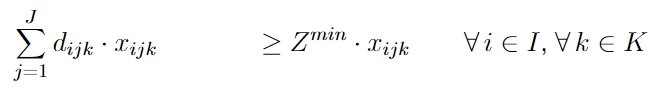•Community ModeratorLooking from afar it seems to me that there is a problem with your $$j$$ index - perhaps the for-loop is in the wrong place?

Could you show the mathematical formulation of the constraint you would like to implement?

Best regards
Jonasz

•Jonasz Staszek that is the mathematical formulation. Maybe I could also just say >= Z I am not really sure about that. What I want is that no durations that are made at once (so on one specific combination of (i,j,k) ) are shorter than the value of Z. Thanks

•Community ModeratorHow do you know which $$j$$ index is to be used for the $$x$$-variable on the right-hand side of your constraint? This is probably the reason for your difficulties.

I am not sure if I understood the context of your problem correctly, but it seems to me that the right-hand side of your inequality should be just $$Z^{min}$$. You will then ensure that the same right-hand side term is applied to all the constraints which you mention.

Best regards
Jonasz

•If I write just Z^min for the RHS, how can I avoid the case for the tuple x beeing 0, than the statement will be 0 >= Z^min which will be false so the model will be infeasible. I tried to do something like

m.addConstrs(quicksum(durations[i,j,k]*x[i,j,k] for j in types for i in stations for k in tasks) >= Z_min for x[i,j,k] != 0)

That doesnt work and gives syntax error. The problem is that x is a tupledict and I dont want the tupledict itself but only the value on the specific points. How can I tell the program that the constraint is only valid when x is not 0